# Stochastic process with independent increments

(diff) ← Older revision | Latest revision (diff) | Newer revision → (diff)

A stochastic process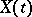such that for any natural numberand all real, the incrementsare mutually-independent random variables. A stochastic process with independent increments is called homogeneous if the probability distribution of,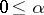,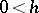, depends only onand not on. Since the result of adding any non-random function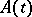tois again a stochastic process with independent increments, the realizations of such processes can be arbitrarily irregular. However, by suitably "centering" the process (say by subtracting fromthe functiondefined by the relation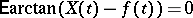), one can make more definite judgements about the structure of the "centred" process. There are at most countably-many (non-random) points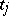at whichhas random jumps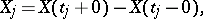and the differenceis a stochastically-continuous stochastic process with independent increments: for anyand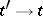,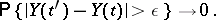A Wiener process and a Poisson process are examples of stochastically-continuous stochastic processes with independent increments (and realizations of the first are continuous with probability one, while realizations of the second are step functions with jumps equal to one). An important example of a stochastic process with independent increments is that of a stable process (cf. Stable distribution). Realizations of a stochastically-continuous stochastic process with independent increments can only have discontinuities of the first kind, with probability one. The distribution of the values of such a process for anyis infinitely divisible (see Infinitely-divisible distribution). In studying stochastic processes with independent increments one can apply the method of characteristic functions (cf. Characteristic function). Problems on the probability of a process crossing a boundary and on the probability distribution of the first crossing time are solved using the so-called factorization identities.

How to Cite This Entry:
Stochastic process with independent increments. Encyclopedia of Mathematics. URL: http://encyclopediaofmath.org/index.php?title=Stochastic_process_with_independent_increments&oldid=18314
This article was adapted from an original article by Yu.V. Prokhorov (originator), which appeared in Encyclopedia of Mathematics - ISBN 1402006098. See original article